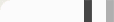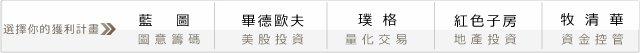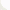再論槓桿空間模型 -- 資金操爆秘訣大公開!

1+[2*k + (n-k)*(-1)]f

(1+[2*k + (n-k)*(-1)]f)^(C(n,k)/2^n)

...

A(f) = (1+[0*2+ (n-0)*(-1)]f)^(C(n,0)/2^n)*
* (1+[1*2+ (n-1)*(-1)]f)^(C(n,1)/2^n)*
* (1+[2*2+ (n-2)*(-1)]f)^(C(n,2)/2^n)*
* (1+[3*2+ (n-3)*(-1)]f)^(C(n,3)/2^n)*
...
* (1+[k*2+ (n-k)*(-1)]f)^(C(n,k)/2^n)*
...
* (1+[(n-1)*2+ (n-(n-1))*(-1)]f)^(C(n,n-1)/2^n)*
* (1+[n*2+ (n-n)*(-1)]f)^(C(n,n)/2^n)

0 意見: